# Ideal Diode and Real Diode

A diode is said to be an Ideal Diode when it is forward biased and acts as a perfect conductor, with zero voltage across it. Similarly, when the diode is reversed biased, it acts as a perfect insulator with zero current through it.

The V-I characteristics of the Ideal diode are shown in the figure below: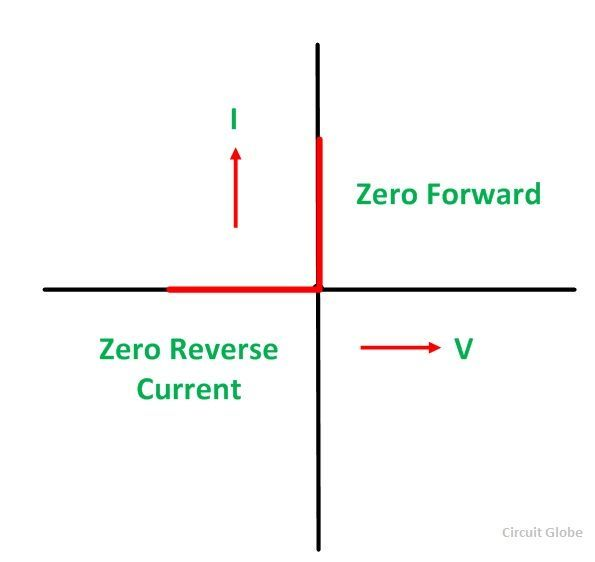An Ideal diode also acts as a switch. When the diode is forward biased it acts like a closed switch as shown in the figure below.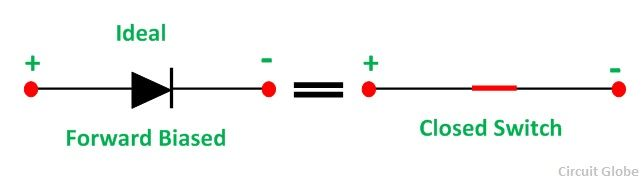Whereas, if the diode is reversed biased, it acts as an open switch as shown in the figure below:

##Real Diode

A Real diode contains barrier potential V0 (0.7 V for silicon and 0.3 V for Germanium) and a forward resistance RF of about 25 ohms. When a diode is forward biased and conducts a forward current IF flows through it which causes a voltage drop IFRF in the forward resistance.

Hence, the forward voltage VF applied across the real diode for conduction, has to overcome the following.

• Potential barrier
• Drop in forward resistance

i.e.,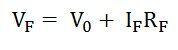For Silicon diode, the equation becomes as shown below:For Silicon diode the equation becomesThe V-I characteristic of the Real diode is shown below: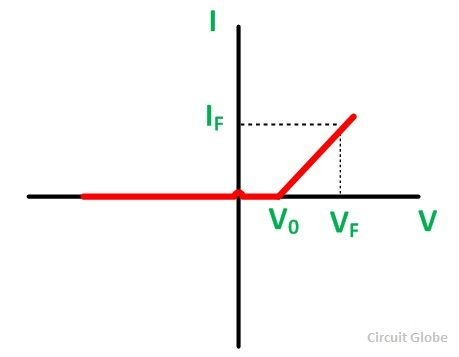For all the practical purposes, a diode is considered to be an open switch when reverse biased. It is because the value of reverse resistance is so high (RR > 100 MΩ) that is considered to be infinite for all practical purposes.

The equivalent circuit of the real diode under forwarding bias condition is shown below:This circuit shows that a real diode still acts as a switch when forward biased, but the voltage required to operate this switch is VF,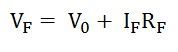This is all about the Ideal Diode.